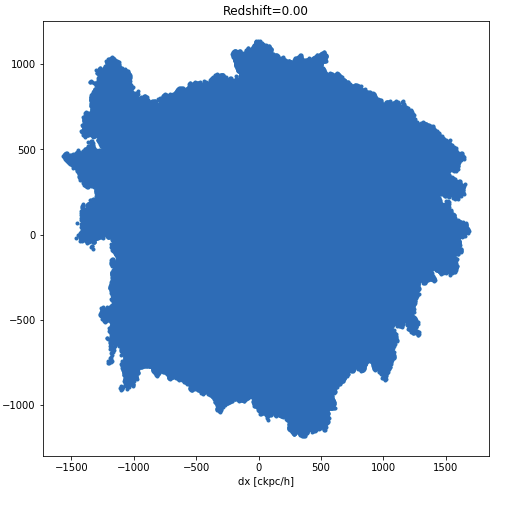### Issue with getting plots for particular elliptical subhalo at certain redshiftsNikita Agarwal
• 1
• 1 Dec '21

Hi,

I am trying to find a good elliptical subhalo evolving from redshift z = 0.4 to z = 0 for a project in the TNG100-1 catalog.

I used the merger tree algorithm to find descendants for a particular subhalo.

``````   tree = il.sublink.loadTree(basePath, 99,60731, fields=['SnapNum','SubfindID','SubhaloPos'],onlyMPB=True)
for i in (72,78,84,91,99):
ind_in_tree = np.where(tree['SnapNum']==i)
print(tree['SubfindID'][ind_in_tree])

Result:





``````

However, while plotting the figure with the script:

``````snap = [72,78,84,91,99]
subhalo_id =[32171,34802,45684,60821,60731]
for (i,j) in zip(snap,subhalo_id):
gas = il.snapshot.loadSubhalo(basePath, i,j, 'gas', fields=None)
``````

I only get the output plot for subhalo_id 60731. I am sure there is nothing wrong with my code as it works for test subhalos.

Regards,
NikitaDylan Nelson
• 1 Dec '21

Dear Nikita,

I don't see that the code you posted produces any output, i.e. I am not sure what output is expected.

If possible please post a minimal reproducible example and I will try to help further.Nikita Agarwal
• 1
• 2 Dec '21

Dear Dylan,

``````basePath = 'sims.TNG/TNG100-1/output'
snap = [72,78,84,91,99]
subhalo_id = [1729364,2058358,11568,16384,17185]

for (i,j) in zip(snap,subhalo_id):
gas = il.snapshot.loadSubhalo(basePath, i, j, 'gas', fields=None)

if ('Coordinates' in gas.keys() ):

gas_pos =(gas['Coordinates'])
sub_pos = subhalo['SubhaloPos']

dx = gas_pos[:,0] -  sub_pos
dy = gas_pos[:,1] -  sub_pos
dz = gas_pos[:,2] -  sub_pos

fig, ax = plt.subplots(figsize=[8,8])
ax.set_xlabel('dx [ckpc/h]')
ax.set_ylabel('dz [ckpc/h]')
print(np.min(dx))
ax.plot(dx, dz, '.')
``````

I expect the output for snaps 72,78,84,91 and 99. However, only get the plot for snap 99.Regards,
NikitaDylan Nelson
• 2 Dec '21

You need to create more than one plot in matplotlib. You could use subplots. If you aren't familiar with matplotlib, I would suggest a getting started tutorial.Nikita Agarwal
• 1
• 2 Dec '21

Hi Dylan,
Thanks, I figured out the error.

The fields for gas.keys() in the above code for snaps [72,78,84,91,99] are as:

``````dict_keys(['count'])
dict_keys(['count'])
dict_keys(['count'])
dict_keys(['count'])
dict_keys(['count', 'CenterOfMass', 'Coordinates', 'Density', 'ElectronAbundance', 'EnergyDissipation', 'GFM_AGNRadiation', 'GFM_CoolingRate', 'GFM_Metallicity', 'GFM_Metals', 'GFM_MetalsTagged', 'GFM_WindDMVelDisp', 'GFM_WindHostHaloMass', 'InternalEnergy', 'InternalEnergyOld', 'Machnumber', 'MagneticField', 'MagneticFieldDivergence', 'Masses', 'NeutralHydrogenAbundance', 'ParticleIDs', 'Potential', 'StarFormationRate', 'SubfindDMDensity', 'SubfindDensity', 'SubfindHsml', 'SubfindVelDisp', 'Velocities'])
``````Dylan Nelson
• 2 Dec '21

Hi Nikita,

That is also true. Perhaps best to check if the subhalo has any gas, e.g.

``````for (i,j) in zip(snap,subhalo_id):
Note also you should always specify `fields` to be just the fields you actually need, this will speed up loading.